﻿ 基于个体时序列差异网络探测疾病恶化的预警信号 Detecting the Early Warning Signals of Disease Progression Based on Individual Difference Time Series Network

Vol. 08  No. 01 ( 2019 ), Article ID: 28656 , 10 pages
10.12677/AAM.2019.81018

Detecting the Early Warning Signals of Disease Progression Based on Individual Difference Time Series Network

Xiaoling Guan

School of Mathematics, South China University of Technology, Guangzhou Guangdong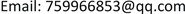Received: Jan. 2nd, 2019; accepted: Jan. 21st, 2019; published: Jan. 28th, 2019ABSTRACT

Pre-disease state is a critical stage of the disease state. Patients in this state can return to the normal state as long as they receive reasonable and effective treatment. Therefore, it is of great significance for medical workers and patients to detect the pre-disease state. In this paper, an algorithm is developed to establish the sampling-specific temporal differential network (SSDN) based on the individual single sample, and according to the sampling-specific temporal differential network, the signal of disease progression can be detected effectively. The validity of the method is verified by numerical simulation and two real data.

Keywords:Single Sample, Temporal Differential Network, Dynamic Network Biomarkers, Composite Variable, Critical-Point1. 引言

2. 方法

2.1. 个体差异性时序网络的构造

1) 构造个体特异网络(Sampling-specific differential network, SSN) (图1(a))。首先，利用疾病数据，选定适量正常的样本作为参考样本，并利用样本的两两基因间的皮尔逊相关系数，作为两个基因节点的边  ，构造参考样本网络。其次，通过把单个样本加到参考样本里，通过两两基因间的皮尔逊相关系数构造扰动样本网络。最后，对比参考网络和扰动网络，根据z-score，保留两个网络共有的具有显著表达的边，进而得到个体特异网络  。

2) 构造个体时序列差异网络(图1(b))。对比两两相邻时间点的个体特异网络，保留它们的差异边，得到个体差异性时序网络(Sampling-specific temporal differential network, SSDN)。

3) 由步骤二，得到个体时序列差异网络(图1(c))，结合差异网络中边和网络节点的特性，用一个复合变量检测出复杂疾病发生病变的临界点。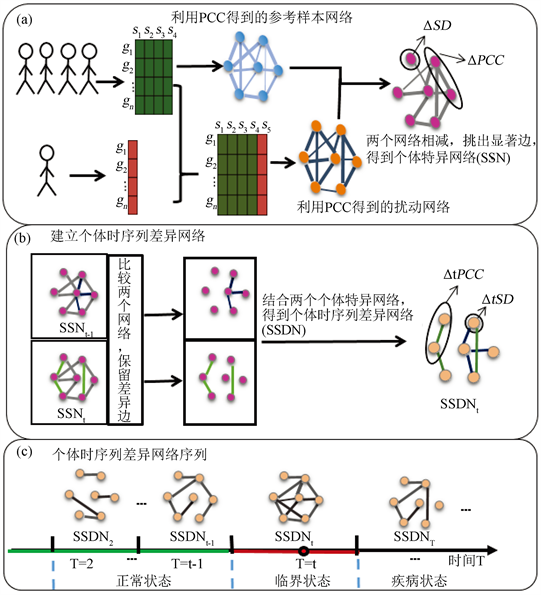Figure 1. Flow chart of construction of sample-specific temporal differential network

2.2. 基于动态网络标志物的复合变量

1) 单样本动态网络标志物中两两元素所构成的个体时序列差异网络边的皮尔逊相关系数的绝对值

2) 单样本动态网络标志物中任意元素的表达方差的差的绝对值( $\Delta tSD$ )的大幅增加。

$I=\left(\frac{\sum \Delta tPCC}{N}\right)\left(\stackrel{¯}{\sum \Delta tSD}\right)$

3. 主要结果

3.1. 八个节点的仿真数据

$\left\{\begin{array}{l}\frac{\text{d}{z}_{1}\left(t\right)}{\text{d}t}=\frac{\left(6-4|P|\right){z}_{2}\left(t\right)}{15\left(1+{z}_{2}\left(t\right)\right)}-\frac{4|P|+3}{15}{z}_{1}\left(t\right)+{\varsigma }_{1}\left(t\right)\\ \frac{\text{d}{z}_{2}\left(t\right)}{\text{d}t}=\frac{\left(3-2|P|\right){z}_{1}\left(t\right)}{15\left(1+{z}_{1}\left(t\right)\right)}-\frac{2|P|+6}{15}{z}_{2}\left(t\right)+{\varsigma }_{2}\left(t\right)\\ \frac{\text{d}{z}_{3}\left(t\right)}{\text{d}t}=-\frac{4|P|-8}{15}+\frac{4-2|P|}{15\left(1+{z}_{1}\left(t\right)\right)}+\frac{4-2|P|}{15\left(1+{z}_{2}\left(t\right)\right)}+{\varsigma }_{3}\left(t\right)\\ \frac{\text{d}{z}_{4}\left(t\right)}{\text{d}t}=-\frac{4|P|-10}{15}+\frac{5-2|P|}{15\left(1+{z}_{1}\left(t\right)\right)}+\frac{5-2|P|}{15\left(1+{z}_{2}\left(t\right)\right)}-{z}_{4}\left(t\right)+{\varsigma }_{4}\left(t\right)\\ \frac{\text{d}{z}_{5}\left(t\right)}{\text{d}t}=-\frac{2}{3}+\frac{2}{15\left(1+{z}_{1}\left(t\right)\right)}+\frac{2}{15\left(1+{z}_{2}\left(t\right)\right)}+\frac{2}{5\left(1+{z}_{3}\left(t\right)\right)}-\frac{6}{5}{z}_{5}\left(t\right)+{\varsigma }_{5}\left(t\right)\\ \frac{\text{d}{z}_{6}\left(t\right)}{\text{d}t}=-\frac{7}{15}+\frac{1}{15\left(1+{z}_{1}\left(t\right)\right)}+\frac{1}{15\left(1+{z}_{2}\left(t\right)\right)}+\frac{1}{5\left(1+{z}_{3}\left(t\right)\right)}+\frac{1}{5\left(1+{z}_{5}\left(t\right)\right)}\\ \text{}+\frac{1}{5\left(1+{z}_{7}\left(t\right)\right)}+\frac{{z}_{8}\left(t\right)}{5\left(1+{z}_{8}\left(t\right)\right)}-\frac{7}{5}{z}_{6}\left(t\right)+{\varsigma }_{6}\left(t\right)\\ \frac{\text{d}{z}_{7}\left(t\right)}{\text{d}t}=\frac{{z}_{8}\left(t\right)}{10\left(1+{z}_{8}\left(t\right)\right)}-\frac{17}{10}{z}_{7}\left(t\right)+{\varsigma }_{7}\left(t\right)\\ \frac{\text{d}{z}_{8}\left(t\right)}{\text{d}t}=\frac{{z}_{7}\left(t\right)}{10\left(1+{z}_{7}\left(t\right)\right)}-\frac{17}{10}{z}_{8}\left(t\right)+{\varsigma }_{8}\left(t\right)\end{array}$ (1)

$\left\{\begin{array}{l}{z}_{1}\left(k+1\right)={z}_{1}\left(k\right)+\left[\frac{\left(6-4|P|\right){z}_{2}\left(k\right)}{15\left(1+{z}_{2}\left(k\right)\right)}-\frac{4|P|+3}{15}{z}_{1}\left(k\right)+{\varsigma }_{1}\left(k\right)\right]\Delta t\\ {z}_{2}\left(k+1\right)={z}_{2}\left(k\right)+\left[\frac{\left(3-2|P|\right){z}_{1}\left(k\right)}{15\left(1+{z}_{1}\left(k\right)\right)}-\frac{2|P|+6}{15}{z}_{2}\left(k\right)+{\varsigma }_{2}\left(k\right)\right]\Delta t\\ {z}_{3}\left(k+1\right)={z}_{3}\left(k\right)+\left[-\frac{4|P|-8}{15}+\frac{4-2|P|}{15\left(1+{z}_{1}\left(k\right)\right)}+\frac{4-2|P|}{15\left(1+{z}_{2}\left(k\right)\right)}+{\varsigma }_{3}\left(k\right)\right]\Delta t\\ {z}_{4}\left(k+1\right)={z}_{4}\left(k\right)+\left[-\frac{4|P|-10}{15}+\frac{5-2|P|}{15\left(1+{z}_{1}\left(k\right)\right)}+\frac{5-2|P|}{15\left(1+{z}_{2}\left(k\right)\right)}-{z}_{4}\left(k\right)+{\varsigma }_{4}\left(k\right)\right]\Delta t\\ {z}_{5}\left(k+1\right)={z}_{5}\left(k\right)+\left[-\frac{2}{3}+\frac{2}{15\left(1+{z}_{1}\left(k\right)\right)}+\frac{2}{15\left(1+{z}_{2}\left(k\right)\right)}+\frac{2}{5\left(1+{z}_{3}\left(k\right)\right)}-\frac{6}{5}{z}_{5}\left(k\right)+{\varsigma }_{5}\left(k\right)\right]\Delta t\\ {z}_{6}\left(k+1\right)={z}_{6}\left(k\right)+\left[-\frac{7}{15}+\frac{1}{15\left(1+{z}_{1}\left(k\right)\right)}+\frac{1}{15\left(1+{z}_{2}\left(k\right)\right)}+\frac{1}{5\left(1+{z}_{3}\left(k\right)\right)}+\frac{1}{5\left(1+{z}_{5}\left(k\right)\right)}\\ \text{}+\frac{1}{5\left(1+{z}_{7}\left(k\right)\right)}+\frac{{z}_{8}\left(k\right)}{5\left(1+{z}_{8}\left(k\right)\right)}-\frac{7}{5}{z}_{6}\left(k\right)+{\varsigma }_{6}\left(k\right)\right]\Delta t\\ {z}_{7}\left(k+1\right)={z}_{7}\left(k\right)+\left[\frac{{z}_{8}\left(k\right)}{10\left(1+{z}_{8}\left(k\right)\right)}-\frac{17}{10}{z}_{7}\left(k\right)+{\varsigma }_{7}\left(k\right)\right]\Delta t\\ {z}_{8}\left(k+1\right)={z}_{8}\left(k\right)+\left[\frac{{z}_{7}\left(k\right)}{10\left(1+{z}_{7}\left(k\right)\right)}-\frac{17}{10}{z}_{8}\left(k\right)+{\varsigma }_{8}\left(k\right)\right]\Delta t\end{array}$ (2)

$\Delta t$ 表示微小的时间间隔，注意到 $Z\left(k\right)$$Z\left(t\right)$$k\Delta t$ 时刻的向量。我们把差分系统(1)的雅可比矩阵用 $J={\frac{\partial f\left(Z\left(k\right);P\right)}{\partial Z}|}_{Z=\stackrel{¯}{Z}}$ 表示，其中

$J={\text{e}}^{\Delta t\cdot A}$ (3)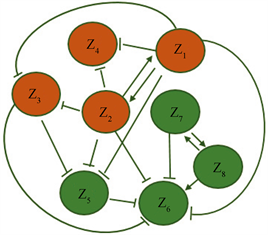Figure 2. Eight gene regulatory networks and their differential equations

$E=\left(-\frac{2}{5}|P|,-\frac{3}{5},-\frac{4}{5},-1,-\frac{6}{5},-\frac{7}{5},-\frac{8}{5},-\frac{9}{5}\right)$

$S=\left[\begin{array}{cccccccc}-2& 1& 0& 0& 0& 0& 0& 0\\ -1& -1& 0& 0& 0& 0& 0& 0\\ 1& 0& -1& 0& 0& 0& 0& 0\\ 1& 0& 0& -1& 0& 0& 0& 0\\ 0& 0& 1& 0& -1& 0& 0& 0\\ 0& 0& 0& 0& 1& -1& 0& 1\\ 0& 0& 0& 0& 0& 0& -1& 1\\ 0& 0& 0& 0& 0& 0& -1& -1\end{array}\right]$

$\begin{array}{l}{z}_{1}-\stackrel{¯}{{z}_{1}}=-2{y}_{1}-{y}_{2}\\ {z}_{2}-\stackrel{¯}{{z}_{2}}=-{y}_{1}-{y}_{2}\\ {z}_{3}-\stackrel{¯}{{z}_{3}}={y}_{1}-{y}_{3}\\ {z}_{4}-\stackrel{¯}{{z}_{4}}={y}_{1}-{y}_{4}\\ {z}_{5}-\stackrel{¯}{{z}_{5}}={y}_{3}-{y}_{5}\\ {z}_{6}-\stackrel{¯}{{z}_{6}}={y}_{5}-{y}_{6}+{y}_{8}\\ {z}_{7}-\stackrel{¯}{{z}_{7}}=-{y}_{7}-{y}_{8}\\ {z}_{8}-\stackrel{¯}{{z}_{8}}=-{y}_{7}-{y}_{8}\end{array}$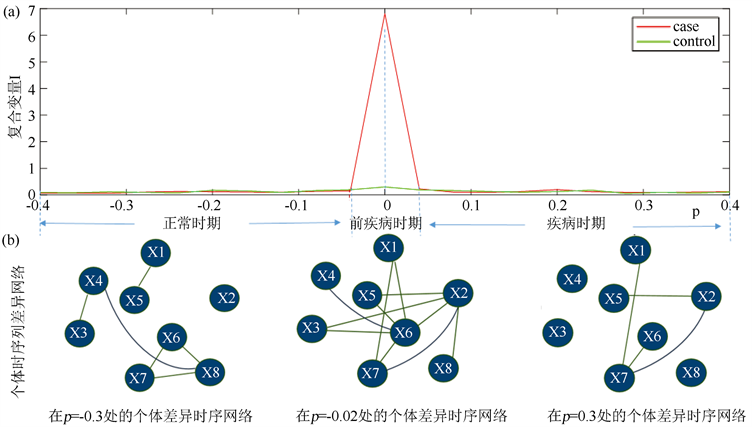Figure 3. The line chart of numerical simulation and the sample-specific temporal differential network

3.2. 方法应用于两个真实的疾病数据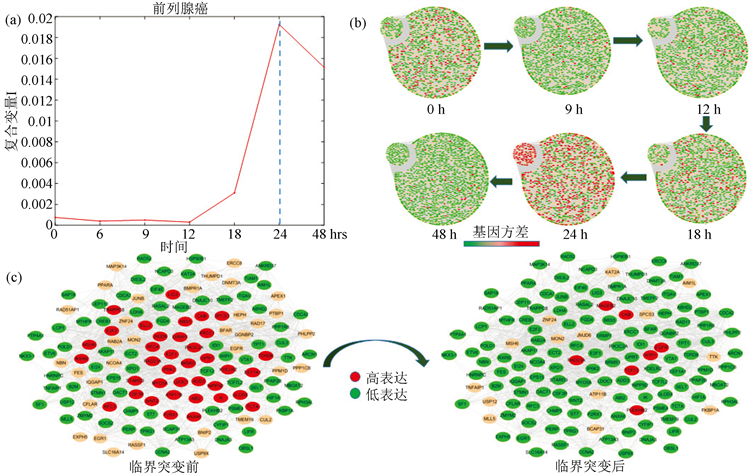Figure 4. The line chart of early-warning signals, the dynamically changes in the network and overturn network during the progression of prostate cancer

4. 讨论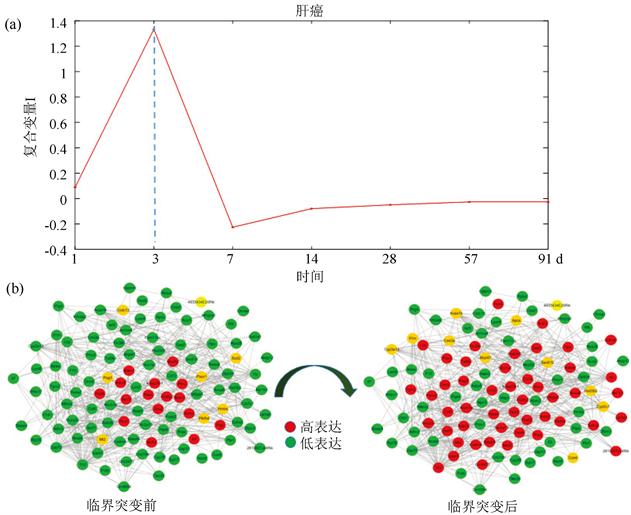Figure 5. The line chart of early-warning signals and the overturn network during the progression of liver cancer

Detecting the Early Warning Signals of Disease Progression Based on Individual Difference Time Series Network[J]. 应用数学进展, 2019, 08(01): 160-169. https://doi.org/10.12677/AAM.2019.81018

1. 1. Liu, X., Wang, Y., Ji, H., et al. (2016) Personalized Characterization of Diseases Using Sample-Specific Networks. Nucleic Acids Research, 44, e164. https://doi.org/10.1093/nar/gkw772

2. 2. Pei, C., Yongjun, Li., Liu, X., Liu, R. and Luonan, C. (2017) Detecting the Tipping Points in a Three-State Model of Complex Diseases by Temporal Dif-ferential Networks. Journal of Translational Medicine, 15, 217. https://doi.org/10.1186/s12967-017-1320-7

3. 3. Liu, R., et al. (2012) Identifying Critical Transitions and Their Leading Biomolecular Networks in Complex Diseases. Scientific Reports, 2, 813. https://doi.org/10.1038/srep00813

4. 4. Liu, R., Yu, X., Liu, X., Xu, D., Aihara, K. and Chen, L. (2014) Identifying Critical Transitions of Complex Diseases Based on a Single Sample. Bioinformatics, 30, 1579-1586. https://doi.org/10.1093/bioinformatics/btu084

5. 5. Chen, P. and Li, Y. (2016) The Decrease of Consistence Probability: At the Crossroad of Catastrophic Transition of a Biological System. BMC Systems Biology, 10, 50. https://doi.org/10.1186/s12918-016-0295-y

6. 6. Liu, X.P., Liu, Z.-P., Zhao, X.-M. and Chen, L.N. (2012) Iden-tifying Disease Genes and Module Biomarkers by Differential Interactions. Journal of the American Medical Informatics Association, 19, 241-248. https://doi.org/10.1136/amiajnl-2011-000658

7. 7. Chen, L., Liu, R., Liu, Z., Li, M. and Aihara, K. (2012) De-tecting Early-Warning Signals for Sudden Deterioration of Complex Diseases by Dynamical Network Biomarkers. Scientific Reports, 2, 342. https://doi.org/10.1038/srep00342

8. 8. Louro, R., Nakaya, H.I., Amaral, P.P., et al. (2007) Androgen Responsive Intronic Non-Coding RNAs. BMC Biology, 5, 4. https://doi.org/10.1186/1741-7007-5-4

9. 9. Lempiäinen, H., Couttet, P., Bolognani, F., et al. (2013) Identification of Dlk1-Dio3 Imprinted Gene Cluster Noncoding RNAs as Novel Candidate Biomarkers for Liver Tumor Promotion. Toxicological Sciences, 131, 375-386. https://doi.org/10.1093/toxsci/kfs303